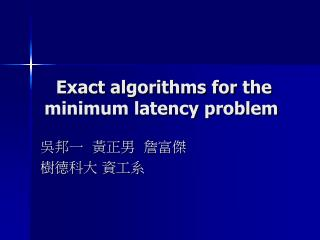Download PresentationExact algorithms for the minimum latency problem

# Exact algorithms for the minimum latency problem - PowerPoint PPT PresentationDownload Presentation## Exact algorithms for the minimum latency problem

- - - - - - - - - - - - - - - - - - - - - - - - - - - E N D - - - - - - - - - - - - - - - - - - - - - - - - - - -
##### Presentation Transcript

1. Exact algorithms for the minimum latency problem 吳邦一 黃正男 詹富傑 樹德科大 資工系

2. P2 10 100 6 P1 5 Routing in a network Which is better, P1 or P2?

3. P2 10 100 6 P1 5 Routing in a network

4. Routing in a network • System’s aspect • Minimize the total length • Traveling salesperson problem • Customers’ aspect • Minimize the total delay • The minimum latency problem

5. Complexity and approximability • Polynomial-time solvable • Path, unweighted tree, tree of diameter 3, tree with constant leaves • NP-hard even for weighted tree • Approximation ratios • (STOC 94)144 → 21.5 → 10.8 → 3.59 (FOCS 03)

6. Our result • Exact algorithms using a technique combining the advantages of both Dynamic Programming and Branch-and-Bound • Experimental results on random and real data

7. Dynamic programming • Solution space: all permutations • (n-1)! (except the starting node) • Configuration (S,r) of a subtour P • S: the visited node, i.e., V(P) • r: the last node of P • Only one subtour for each configuration is needed, the best one but not the shortest one. • Only O(n2^n) configurations

8. Dynamic programming Configurations for k visited nodes Configurations for k+1 visited nodes

9. Branch-and-bound • A tree searching algorithm • A lower bound function for each subtour • A global upper bound for the optimal (a feasible solution) • A subtour with lower bound >= UB is useless subtour P All possible successive nodes extend P by one node

10. Dynamic programming with pruning Configurations for k visited nodes If lower bound <UB, keep If lower bound >=UB, discard Configurations for k+1 visited nodes

11. What we need • A global UB • A greedy method • A lower bound function • A series of lower bound functions Bk(P) • Preprocessing time: O(nk+1) • For each subtour: O(kn) time • Trade-off between quality and time • A data structure to store the configurations

12. Configurations for k visited nodes If lower bound <UB, keep If lower bound >=UB, discard Configurations for k+1 visited nodes How to search a configuration How to search?

13. In a traditional DP • Encoding each configuration into an integer • Using a BIG array • O(1) for searching but the whole array needs to be scanned. It’s just what we want to avoid • An AVL tree is employed.

14. Experiments: random data

15. Experiments: real dataTSPLIB Perform much better than on random data

16. Discussion • A good modification for DP and B&B • Using a good data structure such as the AVL tree is very important. • For small j>i, DPP(j) is better than DPP(i) when n is sufficiently large. • Theoretically, we have more precise lower bound functions. But it suffers from a long computation time and therefore has a worse performance. • In fact, we have tried several other lower bound functions. Some of them eliminate much more subtours than B1 but has a worse performance

17. Thank you